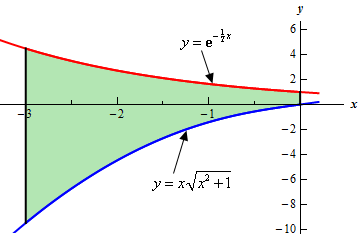Paul's Online Notes
Home / Calculus I / Applications of Integrals / Area Between Curves
Show Mobile Notice Show All Notes Hide All Notes
Mobile Notice
You appear to be on a device with a "narrow" screen width (i.e. you are probably on a mobile phone). Due to the nature of the mathematics on this site it is best views in landscape mode. If your device is not in landscape mode many of the equations will run off the side of your device (should be able to scroll to see them) and some of the menu items will be cut off due to the narrow screen width.

### Section 6.2 : Area Between Curves

7. Determine the area of the region bounded by $$y = x\sqrt {{x^2} + 1}$$, $$y = {{\bf{e}}^{ - \,\,\frac{1}{2}x}}$$, $$x = - 3$$ and the $$y$$-axis.

Show All Steps Hide All Steps

Hint : It’s generally best to sketch the bounded region that we want to find the area of before starting the actual problem. Having the sketch of the graph will usually help with determining the upper/lower functions and the limits for the integral.
Start Solution

Let’s start off with getting a sketch of the region we want to find the area of.

Note that using a graphing calculator or computer may be needed to deal with the first equation, however you should be able to sketch the graph of the second equation by hand.

Here is a sketch of the bounded region we want to find the area of.Show Step 2

It should be clear from the graph that the upper function is $$y = {{\bf{e}}^{ - \,\,\frac{1}{2}x}}$$ and the lower function is $$y = x\sqrt {{x^2} + 1}$$.

Next, we were given limits on $$x$$ in the problem statement (recall that the $$y$$-axis is just the line $$x = 0$$!) and we can see that the two curves do not intersect in that range. Note that this is something that we can’t always guarantee and so we need the graph to verify if the curves intersect or not. We should never just assume that because limits on $$x$$ were given in the problem statement that the curves will not intersect anywhere between the given limits.

So, because the curves do not intersect we will be able to find the area with a single integral using the limits : $$- 3 \le x \le 0$$.

Show Step 3

At this point there isn’t much to do other than step up the integral and evaluate it.

We are assuming that you are comfortable with basic integration techniques, including substitution since that will be needed here, so we’ll not be including any discussion of the actual integration process here and we will be skipping some of the intermediate steps.

The area is,

$A = \int_{{ - 3}}^{0}{{{{\bf{e}}^{ - \,\,\frac{1}{2}x}} - x\sqrt {{x^2} + 1} \,dx}} = \left. {\left( { - 2{{\bf{e}}^{ - \,\,\frac{1}{2}x}} - \frac{1}{3}{{\left( {{x^2} + 1} \right)}^{\frac{3}{2}}}} \right)} \right|_{ - 3}^0 = \require{bbox} \bbox[2pt,border:1px solid black]{{ - \frac{7}{3} + 2{{\bf{e}}^{\frac{3}{2}}} + \frac{1}{3}{{10}^{\frac{3}{2}}} = 17.17097}}$